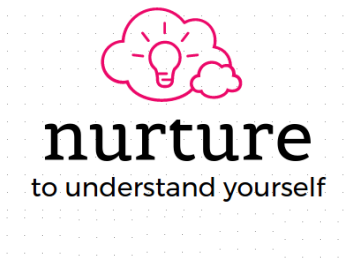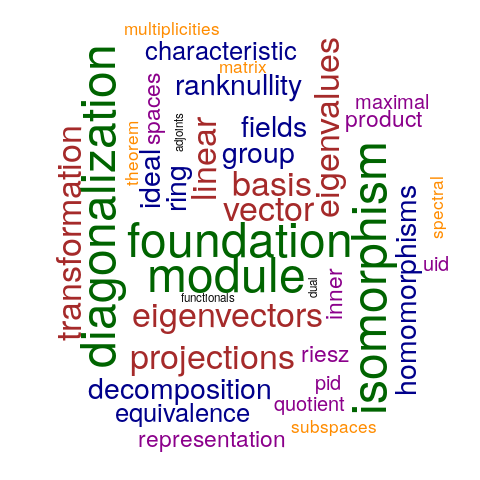Pedagogical knowledge of Mathematics
The teaching of Linear Algebra
18th - 22nd December 2017
Central University of Tamil Nadu

This workshop will aim to touch upon some of these topics

Foundation

Vector Spaces, Subspaces, Basis, Linear transformation, Quotient Space, Rank-nullity theorem, Matrix representations, Inner product Spaces

Diagonalization

Eigenvalues and Eigenvectors; Geometric and Algebraic multiplicities, characteristic equation, spectral theorem for a symmetric operator in a f.d. inner
product space. Structure of a single linear transformation, Algebra of Projections, Equivalence of Matrices and Similarity of Matrices

Isomorphism Theorems

Isomorphism theorems, Linear Functionals, Dual bases, Operator Adjoints, norm, orthogonal direct sums,The Riesz Representation Theorem

Algebraic Structures

Groups, Subgroups, Ring, Ideals, Quotient Rings, Maximal Ideals, Quotient fields, PIDs, UIDs, Fields and Algebras

Module

Modules, Submodules, Homomorphisms , Quotient Modules, The Cyclic Decomposition of a Primary Module

Workshop Cloud